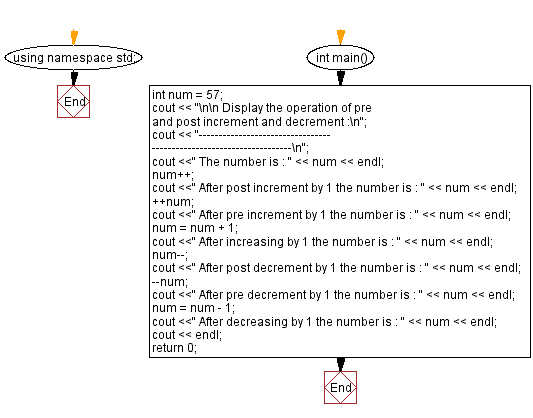﻿ C++ : Operation of pre and post increment and decrement

# C++ Exercises: Display the operation of pre and post increment and decrement

## C++ Basic: Exercise-9 with Solution

Write a C++ program to display the operation of pre and post increment and decrement.

Sample Solution:

C++ Code :

``````#include <iostream>
using namespace std;

int main()
{
int num = 57;
cout << "\n\n Display the operation of pre and post increment and decrement :\n";
cout << "--------------------------------------------------------------------\n";
cout <<" The number is : " << num << endl;
num++;               // increase by 1 (post-increment)
cout <<" After post increment by 1 the number is : " << num << endl;
++num;               // increase by 1 (pre-increment)
cout <<" After pre increment by 1 the number is : " << num << endl;
num = num + 1;  // num is now increased by 1.
cout <<" After increasing by 1 the number is : " << num << endl; // 79
num--;               // decrease by 1 (post-decrement)
cout <<" After post decrement by 1 the number is : " << num << endl;
--num;               // decrease by 1 (pre-decrement)
cout <<" After pre decrement by 1 the number is : " << num << endl;
num = num - 1; // num is now decreased by 1.
cout <<" After decreasing by 1 the number is : " << num << endl;
cout << endl;
return 0;
}
``````

Sample Output:

``` Display the operation of pre and post increment and decrement :
--------------------------------------------------------------------
The number is : 57
After post increment by 1 the number is : 58
After pre increment by 1 the number is : 59
After increasing by 1 the number is : 60
After post decrement by 1 the number is : 59
After pre decrement by 1 the number is : 58
After decreasing by 1 the number is : 57
```

Flowchart:C++ Code Editor:

What is the difficulty level of this exercise?

﻿

## C++ Programming: Tips of the Day

Why is there no std::stou?

The most pat answer would be that the C library has no corresponding "strtou", and the C++11 string functions are all just thinly veiled wrappers around the C library functions: The std::sto* functions mirror strto*, and the std::to_string functions use sprintf.

Ref: https://bit.ly/3wtz2qA

We are closing our Disqus commenting system for some maintenanace issues. You may write to us at reach[at]yahoo[dot]com or visit us at Facebook